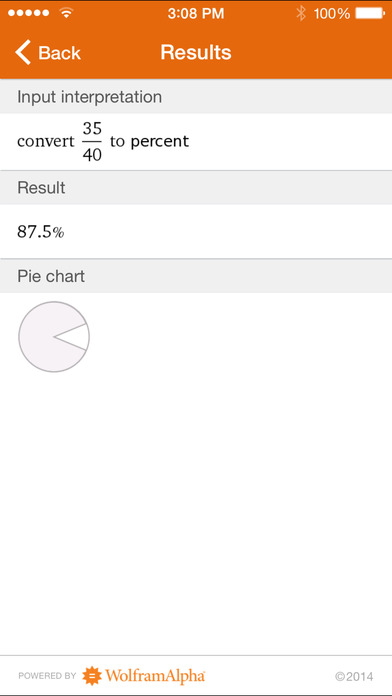Wolfram Fractions Reference App

iOS UniversalReference

\$0.99

Learning fractions? Then you need the Wolfram Fractions Reference App. Whether adding, subtracting, or converting fractions, the Wolfram Fractions Reference App will help you with your specific fraction problems.

- Visualize fractions on a number line or pie chart
- Convert a fraction to a decimal or percent, or vice versa
- Get help with arithmetic including addition, subtraction, multiplication, and division
- Reduce fractions to their simplest forms

The Wolfram Fractions Reference App is powered by the
Wolfram|Alpha computational knowledge engine and is created by Wolfram Research, makers of Mathematica—the world's leading software system for mathematical research and education.

The Wolfram Fractions Reference App draws on the computational power of Wolfram|Alpha's supercomputers over a 3G, 4G, or Wi-Fi connection.

What's New

- Bug fixes

•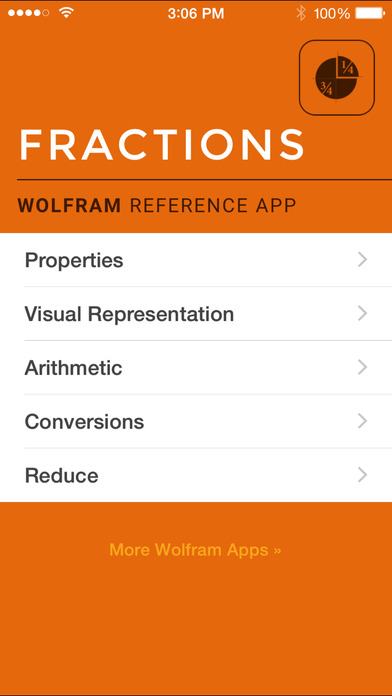•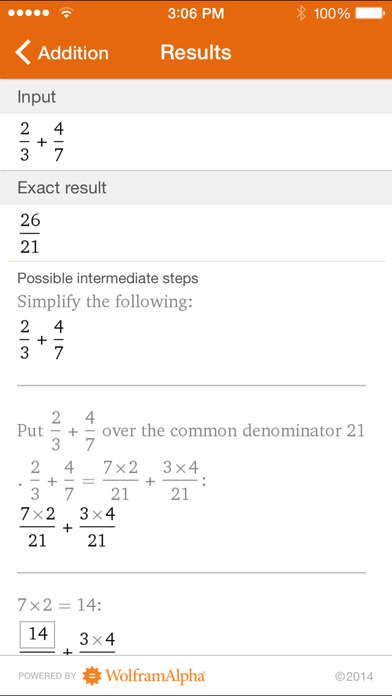•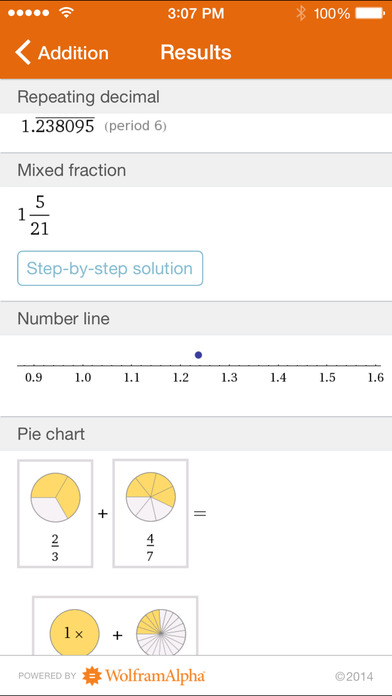•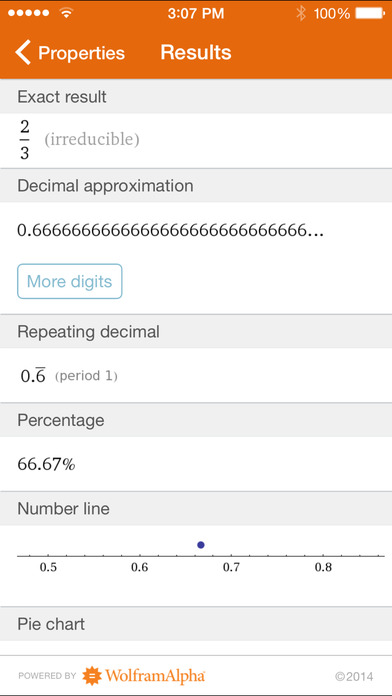•